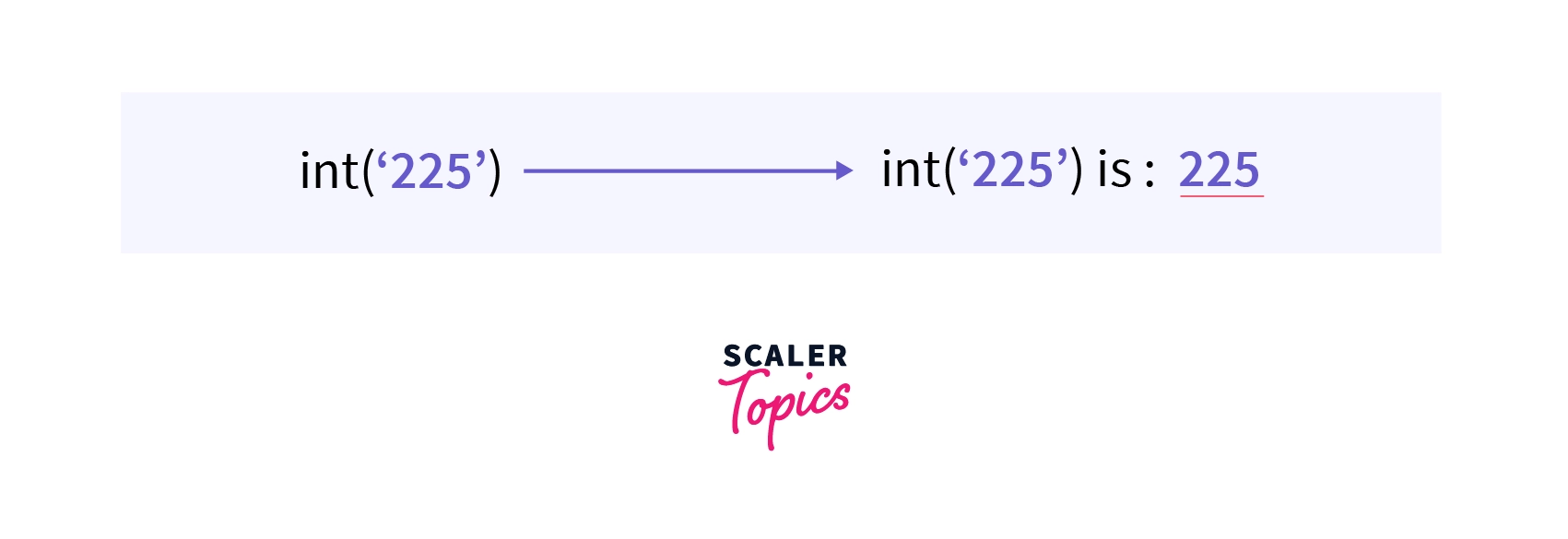# int() Function in Python

Challenge Inside! : Find out where you stand! Try quiz, solve problems & win rewards!
Learn via video coursePython Course for Beginners With Certification: Mastering the Essentials
By Rahul JanghuFree
4.90Enrolled: 1000Python Course for Beginners With Certification: Mastering the Essentials
Rahul JanghuFree
4.90
Enrolled: 1000

## Overview

To convert a specified value (any number or string) into an integer object, we use int() in python. The conversion of one data type into the other data type is known as type casting or type conversion.

## Syntax of int() in Python

The syntax of the method, int() in Python is very simple:

The value parameter is required, but the base parameter is optional.

## Parameters of int() in Python

The method - int() in python takes two parameters, one optional and the other required. The parameters given in int in python are:

1. value: The value parameter is a required parameter. The value is the number or string to be converted into an integer object.
2. base: The base parameter is optional. The default value specified is 10. The base-10 value resembles the number having base address 10, i.e. decimal numbers.

We can specify the base type of the number other than 10, such as

• 8 for octal number (to convert octal number into an equivalent decimal number).
• 16 for hexadecimal number (to convert a hexadecimal number into an equivalent decimal number).
• 2 for binary number (to convert the binary number into an equivalent decimal number).

## Return Values of int() in Python

The int in python returns the integer object value of the given string or floating number. If we do not give any parameter in the method, it will return 0.

## Exception of int() in Python

The int() in python raises an error whenever we pass a data type other than a string or an integer. For example, if we try to typecast a list using int in python. The following error will be printed:

This exception raised by int() in python is known as TypeError. Refer to this article's topic - More Example to know more about the exceptions.

## Example

Let us convert a floating-point number into an integer using the method int() in python.

Output:

## What is int() in Python?

As we know, converting one data type into the other is known as type casting or type conversion.

We can convert any string into an integer using the built-in python method called int() method. The int() method takes a string or integer data type and converts the given data into an integer number.## How to Use int() in Python?

To convert a specified value (any number or string) into an integer object, we use int in python. The int in python is a built-in method that converts a string or a number into an integer. We can also use int in python to convert binary numbers to decimal numbers, hexadecimal numbers to decimal numbers, and octal numbers into decimal numbers.

The int method raises an error when a non-string or non-integer data type is given as a parameter. The error raised is termed as TypeError.

## More Examples

Let us take a few examples to better understand the int method in python.

### Example for Exception in int

Let us try to convert a list into an integer using int in python. As we have learned, we can only convert a string or a number into an integer.

Output:

### How int() works in Python?

Let us try to convert a string into an integer using int in python.

Output:

### How int() works for binary, octal, and hexadecimal?

Let us try to convert a binary, octal, and hexadecimal number into integer numbers using int in python.

Output

### int() for custom objects

Let us try to convert an object called Student into an integer number using int in python.

Output:

Note:

Internally the int in python calls int() method, which is the method of an object. So we can convert an object into an integer object using int in python.

We only need to override the int() method for a class to return an integer class.

## Conclusion

• To convert a specified value (any number or string) into an integer object, we use int in python.
• The conversion of one data type into the other is known as type casting or type conversion.
• The int() in python takes two parameters and returns an equivalent integer number. Its syntax is: int(value, base)
• The value parameter is required, but the base parameter is optional.
• Whenever we pass a data type other than a string or an integer, the int in python raises an error. The raised error is known as TypeError.